# Exponential Excel FunctionArticle byExcelMojo Team## What Is Exponential In Excel (EXP)?

The Exponential in Excel (EXP) is an inbuilt Math & Trig function. It determines the result of raising the constant e to the power of the specified number. And e is the natural logarithm’s base, with a value of 2.71828. Users can use the Exponential function in Excel when solving expressions containing exponential terms with the base e.

For example, the table below contains the numbers to which we should raise the base e to get the required exponential values according to the given descriptions.

Suppose the requirement is to display the result in column C cell range C2:C6. Then, considering the meaning of Exponential function explained above, applying the EXP() in the target cells can get us the desired outcome.

The EXP() in each target cell accepts the corresponding column B Number value in the above example. And it raises the base e to the power of the specified number to return the required exponential value.

For example, the cell C2 formula accepts the cell B2 value, 5, as the input, and it raises the base e to the power 5 to return 148.4131591 as the Exponential function in Excel output.

###### Key Takeaways
• The Exponential function in Excel computes the base e, the natural logarithm’s base, with a value of 2.71828, raised to the power of the specified number.
• Users can use the EXP function in formulas, representing mathematical expressions containing exponential terms with the base e. For example, the function helps determine the growth or decay rate, an unknown variable’s value, and population growth.
• Then EXP function takes one mandatory argument, number, as input.
• We can use the EXP function with other inbuilt Excel functions, such as ROUND, to achieve fruitful results.

### EXP() Excel Formula

The syntax of the Exponential function in Excel is:

where,

• number: The exponent to which we must raise the base e using Exponential function in Excel.

The argument in the EXP function in Excel is mandatory.

Further, the EXP() aspects below will help us use the function properly.

• The EXP() argument can only be a number (integers, decimals, fractions). Otherwise, the EXP() returns the #VALUE! error. And it considers an empty cell value as 0 to return the exponential value of 1.
• The smallest and the largest values the EXP() can accept as input are -1 x 10308 and 709.782, respectively. For any number outside this range, the EXP() returns the #NUM! error.
• If the EXP() does not recognize the supplied input as a number, it returns the #NAME error.
• The EXP function works only for the base e. We can use the exponential operator, ‘^’, or the POWER() for other base values.

### How To Use EXP Excel Function?

The steps to use the Exponential function in Excel are as follows:

1. First, confirm the specified input data is a valid number.

2. Next, choose the target cell, and enter EXP.

3. Finally, press Enter to view the resulting exponential value.

The following example shows the above mentioned steps in detail.

The table below contains a list of numbers we require to raise the base e to based on the given description.

Suppose the target cells are in the range C2:C6. Then, using Exponential function in Excel, we can obtain the desired exponential values for the cell range C2:C6.

Step 1: First, select the target cell C2, enter the EXP(), and then, press Enter.

=EXP(B2)

We can also enter the value directly as the EXP() argument and get the same output.

=EXP(2.3)

Alternatively, we can choose the target cell C2 and navigate through the path FormulasMath & TrigEXP to apply the function from the Formulas tab.

The above step will open the Function Arguments window.

Once we fill in the Number field in the Function Arguments window, as shown below, and then, click OK, we will see the EXP() executed in the target cell C2.

Step 2: Next, using the fill handle, implement the formula in cell range C3:C6.

Let us consider the cell C6 expression to understand how the formula works. The EXP() accepts the cell B6 value, 1/5, as the number argument. It then calculates the base e raised to the power 1/5 and returns the result as 1.221402758.

### Examples

Check out the following illustrations to understand the meaning of Exponential function in Excel and apply the EXP() in the best way possible.

#### Example #1

The below table contains the parameter values required to determine the continuous compound interest.

Suppose the requirement is to calculate and display the continuous compound interest in cell C8. Then, here is how applying the EXP function in Excel cell C8 will get us the required result.

Step 1: To begin with, select the target cell C8, enter the below formula, and then, press Enter.

=C4*EXP(C5*C6)

The above expression represents the mathematical formula of continuous compound interest provided in cell A1.

So first, the expression inside the Exponential function in Excel cell C8 multiplies the r and t values, 10% and 5, to return the value 0.5. Next, the EXP() finds the base e to the power 0.5, which is 1.64872127. Then the formula multiplies the EXP() output with \$1,000 to return the final amount as \$1,648.72.

#### Example #2

We shall now see how to plot Exponential function in Excel. The following image shows an exponential function in cell B1.

Suppose the value a is 0.02 (refer to cell B2), the x-values are in the table shown in the above image, and the requirement is to determine y-values based on the given x-values.

Assume the target cell range is B5:B14. Then, here is how we can use the EXP() in the target cells and then plot EXP function to get the desired output.

Step 1: First, select the target cell B5. Then, enter the below formula, and press Enter.

=\$B\$2*EXP(-2*A5)

Step 2: Next, apply the above formula in cell range B6:B14 using the fill handle in excel.

Let us see the cell B14 expression to check how the formula executes. First, the expression inside the Exponential function in Excel cell B14 returns the product of -2 and 2 (cell A14 value), -4. Next, the EXP() returns the base e to the power -4, 0.01831563. Finally, the formula returns the product of 0.02 and 0.01831563, 0.000366313.

Step 3: Then, select the cell range A4:B14, go to InsertRecommended Charts Scatter to select the Scatter chart, and finally, click OK.

Step 4: Now, right-click on the horizontal axis and then, pick the Format Axis option from the contextual menu to open the corresponding window.

Step 5: Next, set the Major field as 0.2 in the Format Axis pane to adjust the horizontal axis scale and close the pane.

Step 6: Now, click the Chart Elements option (‘+’) on the right of the chart area and enable the Axis Titles option.

Step 7: Then, update the chart and axis titles by selecting the corresponding elements in the chart area.

Thus, the above Scatter plot graphically represents the given Exponential function in Excel.

#### Example #3

We can use the Exponential function in Excel with other inbuilt functions such as ROUND excel function.

The below table shows a list of numbers in column A. Column B contains the exponential values obtained by using the EXP function, with the input being the numbers specified in the corresponding column A cells.

Suppose the requirement is to get the exponential values, rounded to 0 digits, and display the result in column C cell range C2:C6. Then applying the EXP() with the ROUND() in the target cells will fetch us the required data.

Step 1: To begin with, select the target cell C2, enter the below formula, and then, press Enter.

=ROUND(EXP(A2),0)

Step 2: Next, implement the above formula in cell range C3:C6 using the fill handle.

Let us consider the cell C6 expression to see how the formula works. First, the EXP() determines the base e to the power 1/7, 1.153564995. And then, the ROUND() rounds up the EXP() output to 0 digits, thus returning the value 1.

The second argument in the ROUND() is 0. And so, the EXP function output gets rounded to the nearest integer.

### Important Things To Note

• The Exponential function in Excel works only for the base e.
• Ensure the number we supply as the EXP() argument is between -1 x 10309 and 709.783. Else, the function will return the #NUM! error.
• Ensure we supply only a number (integer, decimal, or fraction) as the EXP function argument. Else, the function return value will be the #VALUE! error. On the other hand, the EXP function counts an empty cell as 0, returning 1 as the exponential value.
• For a supplied value not recognized as a number, the EXP function output is the #NAME error. Else we might also get a typo error.

1. Is there an Exponential function in Excel?

There is an Exponential function in the Formulas tab. We can access the function by selecting FormulasMath & TrigEXP.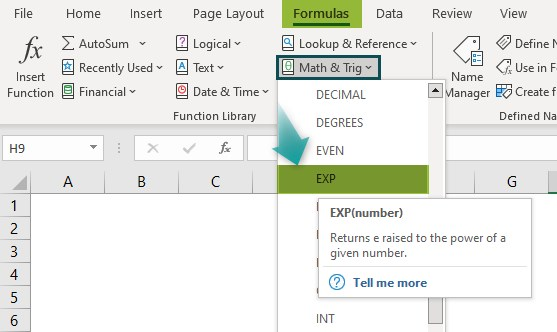2. How to apply the Exponential function in Excel VBA?

We can apply the Exponential function in Excel VBA using the method:

Exp(number)

The argument in the above method has the same definition provided in the EXP() Excel Formula section.

Let us understand how to use the above mentioned method in Excel VBA with an example.

The following table contains a set of numbers.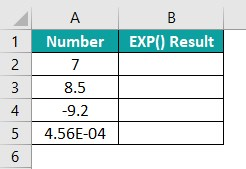Suppose the requirement is to determine the exponential values for the respective numbers provided as EXP() argument and display the results in cells B2:B5. Then, the steps to apply the EXP function in Excel VBA to get the required data in the target cells are as follows:

Step 1: With the active sheet containing the above table open, press the keys Alt + F11 to open the VBA Editor.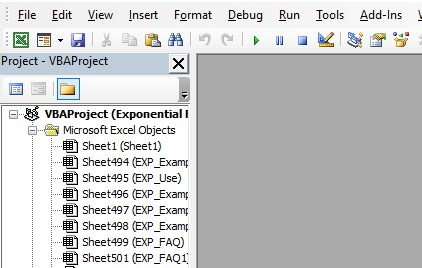Step 2: Next, choose the required VBA Project and then, click InsertModule to access a new module, Module1.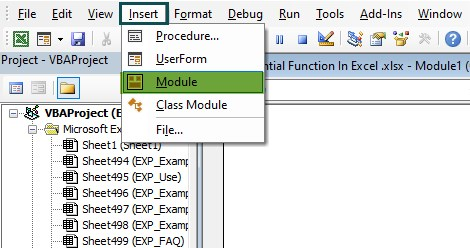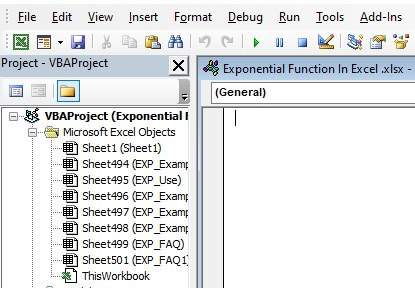Step 3: Now, enter the code in the Module1 window to apply the above mentioned method in the target cells.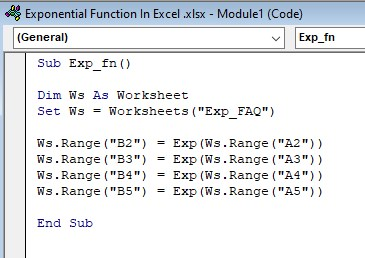Step 4: Next, click the Run Sub/UserForm button to execute the entered code from the top menu.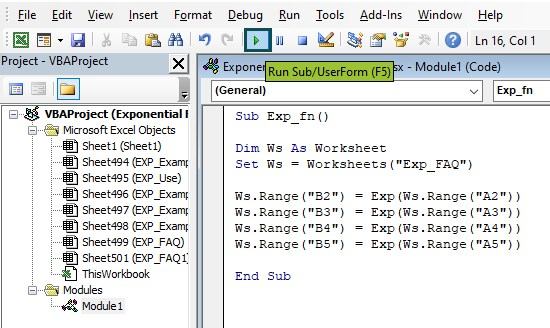Step 5: Then, go to the active worksheet to view the required exponential values in the target cells.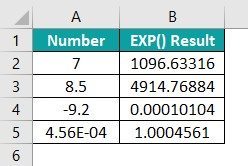Remember, each EXP method in the entered code accepts the corresponding number provided in column A.

And it determines the base e raised to the power of the specified number to display the exponential value is the respective target cell.

Likewise, we can find exponential values in excel.

3. What is the inverse of the Exponential function in Excel?

The inverse of the EXP function in Excel is the LN function.
For example, column A in the below table contains a list of numbers.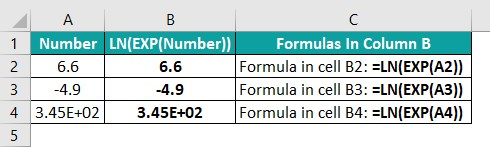And column B shows how applying the EXP() within LN(), with the specified number provided as input, returns the same number, thus proving the inverse of EXP() is the LN().